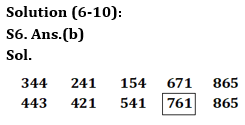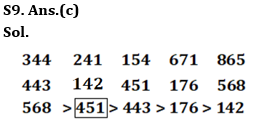Latest Banking jobs   »

# Reasoning Quizzes Quiz For IRDA AM 2023- 20th April

Direction (1-5): Study the following information carefully and answer the questions given below:
a @ 1 8 b d 3 # 4 5 h 7 ! d j 9 % f c \$ g x & d s z n 7 5 @ 8 % h d # j g \$ f x c 6 5 @ d 3 3 !

Q1. How many numbers are there in the series which are immediately followed by a symbol but not immediately preceded by a letter?
(a)One
(b)Two
(c)None
(d)Three
(e)More than three

Q2. Which of the following element is ninth to the left of the tenth element from the right end?
(a)8
(b)j
(c)@
(d)#
(e)None of these

Q3. If all symbols are removed, then which of the following is second to the right of the seventeenth element from the left end?
(a)s
(b)f
(c)9
(d)j
(e)None of these

Q4. How many letters are there in the series which are immediately preceded by a symbol and immediately followed by an odd number?
(a)Three
(b)Two
(c)More than three
(d)One
(e)None

Q5. How many even numbers are there between the elements which are thirteenth from the right end and fourteenth from the left end?
(a)One
(b)None
(c)Two
(d)Three
(e)More than three

Direction (6-10): Following questions are based on the five 3-digit numbers given below, Study the following numbers and answer the following question:
344 241 154 671 865

Q6. If we arrange all the digits in descending order within the number from left to right then which of the following is second highest number?
(a)344
(b)671
(c)241
(d)865
(e)154

Q7. If 1 is added to each odd number and 1 is subtracted from each even number then how many numbers are divisible by 3?
(a)One
(b)None
(c)Two
(d)Three
(e)More than three

Q8. What is the product of third digit of the highest number and the first digit of the second lowest number?
(a)12
(b)10
(c)8
(d)20
(e)None of these

Q9. If the first digit is interchanged with the third digit within the same number, and then the numbers are arranged in decreasing order from left to right then which of the following is second from the left end?
(a)344
(b)241
(c)154
(d)671
(e)865

Q10. The third digit of the third number from the left end is how much more or less than the second digit of the second number from the right end?
(a)1
(b)2
(c)5
(d)4
(e)None of these

Direction (11-15): Study the following series carefully and answer the questions given below:

# \$ ^ 1 2 Y \$ B E ! * % 2 3 ~ V 4 I @ 8 M * U 7 ^ X \$ Z & 6 3 # 4 5 7

Q11. How many symbols are there in the above series which are immediately preceded as well as followed by numbers?
(a) None
(b) Two
(c) One
(d) Three
(e) Four

Q12. Which element is 11th to the right of the element which is 17th from the right end?
(a) 6
(b) &
(c) 3
(d) #
(e) 7

Q13. What is the exact number of vowels between the 5th element from the left end and 14th element from the right end?
(a) None
(b) One
(c) Two
(d) Three
(e) None of these

Q14. What would come at the place of question mark in the following sequence?
#\$1, Y\$E, *%3, ?

(a) %23
(b) MV@
(c) 4M*
(d) V4@
(e) @4%

Q15. Which of the following element is exactly between the 15th element from the left end and 15th element from the right end?
(a) I
(b) @
(c) V
(d) 4
(e) None of these

Solutions

Solution (1-5):
S1. Ans.(e)
Sol. 75@, @8%, 65@, 33!
S2. Ans.(c)
Sol. @
S3. Ans.(a)
Sol. s
S4. Ans.(d)
Sol. @d3
S5. Ans.(a)
Sol. 8S7. Ans.(c)
Sol. 154, 671

S8. Ans.(b)
Sol. 5×2=10S10. Ans.(e)
Sol. 7-4=3

Solutions(11-15):
S11. Ans.(c)
Sol. 3 # 4
S12. Ans.(a)
Sol. 6
S13. Ans.(c)
Sol. E, I
S14. Ans.(d)
Sol. V4@
S15. Ans.(a)
Sol. I## FAQs

### How many sections are there in the IRDAI exam?

There are 4 sections in the IRDAI assistant manager online preliminary exam. They are Reasoning, English Language, General awareness, Quantitative aptitude.

#### Congratulations!Union Budget 2023-24: Free PDF